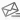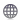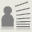# CarlosACoelhocmac@fct.unl.ptHome page
Carlos A. Coelho is Associate Professor, with Habilitation, at the Mathematics Department of Faculdade de Ciências e Tecnologia of Universidade Nova de Lisboa. Ph.D. in Biostatistics by the University of Michigan, and Elected Member of the International Statistical Institute, his main areas of research are Mathematical Statistics and Distribution Theory, namely exact and near-exact distributions for likelihood ratio test statistics used in Multivariate Analysis. Areas of interest are also Estimation, Univariate and Multivariate Linear, Generalized Linear and Mixed Models, as well as Computational Statistics. In a 2004 paper in the Journal of Multivariate Analysis , he laid the foundations for what he called ’near-exact distributions’. Since then these distributions have been successfully applied to a large number of statistics, with more than 20 papers published on this topic. These are distributions which are built using a different philosophy in approximating the exact distribution of statistics. The technique combines the development of an adequate decomposition, most often a factorization, of the characteristic function (c.f.) with the action of then keeping most of this c.f. unchanged, while replacing the remaining smaller part by an adequate asymptotic approximation. All this is done in order to obtain a resulting c.f. that corresponds to a manageable and very well-fitting approximation, which may be used to compute near-exact quantiles or p-values. These distributions are not too hard to obtain and they are much useful in situations where the exact distribution is not possible to obtain in a manageable form. Near-exact distributions may be easily implemented even for test statistics associated with highly complicated hypotheses, by considering the decomposition of the null hypothesis into a set of conditionally independent hypotheses.

Reference:
 Coelho, C. A. (2004). The Generalized Near-Integer Gamma distribution: a basis for ’near-exact’ approximations to the distribution of statistics which are the product of an odd number of independent Beta random variables, Journal of Multivariate Analysis, 89, 191-218.Powered by PublicationsList.org.